# What is a divergence meter for?

In the Steins; Gate anime plot, the divergence meter is a Nixie1 clock/calendar set by the protagonist to show how much an original timeline has been altered by the influences of time travel. In this context, the universe follows lines that converge for some events even though divergences due to the influence of time travel occur. The universe before the invention of a time machine would then diverge 0, since it would follow the course of destiny without interference.

1An electronic device with a wire mesh in the shape of numerals that glow orange when they receive energy, revealing itself inside a glass tube.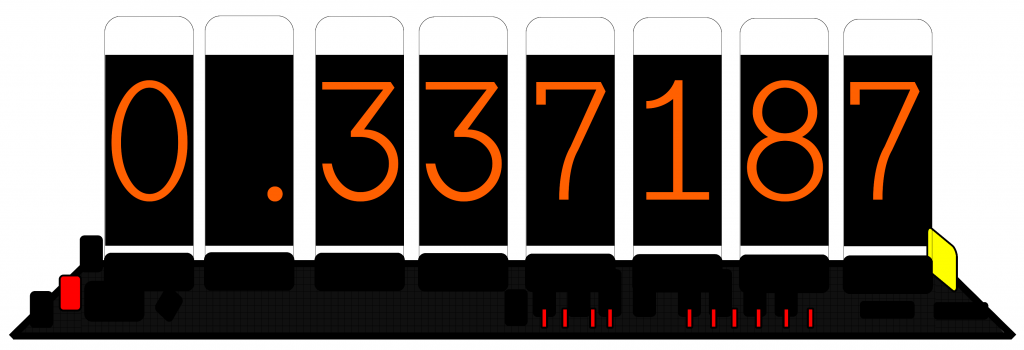We can imagine this behavior similar to the curve of a function that models a real phenomenon. In its ideal scenario, all points of the phenomenon would coincide with the points of the curve that defines this model. However, in a practical scenario (experimental data), we would clearly have points above and below the curve of the model, thus representing errors more or less than what is expected. If the differences are very large, staying in the model becomes unfeasible and we are forced to change to a model that better fits the observed data.

In the example below, I took the function f(x) = |x|3/2 to simulate the universe. Its blue dots are the original events in case the divergence meter is equal to 0, that is, the time machine was not invented. All events exactly match the original curve.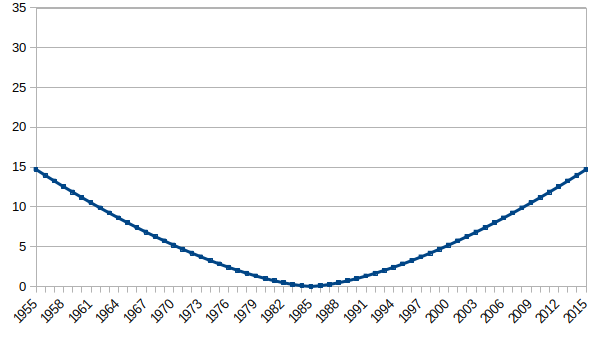Just to situate ourselves in the examples, we will call the arithmetic mean of the errors in module (positive difference between the position of the point and the curve) as the measure of divergence recorded by a divergence meter similar to that of Steins; Gate. For this function, the divergence would be 0, since all the event points coincide exactly with the model.

Similarly, in Steins; Gate even though there are influences of temporal movements that make the divergence meter above 0. As long as the meter is below 1, the model does not change. This affects among other aspects the fate of the characters and events in the plot.

In this same scenario, we can imagine that the time machine was invented and there were small variations resulting from the temporal movement. This consequently generated a divergence greater than 0, but they may not have been so impactful as to require a change of model from the universe. In Steins; Gate’s plot this involved, among other aspects, the preservation of social groups, places, characters, objects. In the next example, I took the function f (x) = |x|3/2 to simulate the universe and a random y between -0,5 and 0,5 to determine the divergences. Thus the divergent points represented in red are described by f(x) = |x+y|3/2, while the model curve represented in blue is described by f (x) = |x|3/2.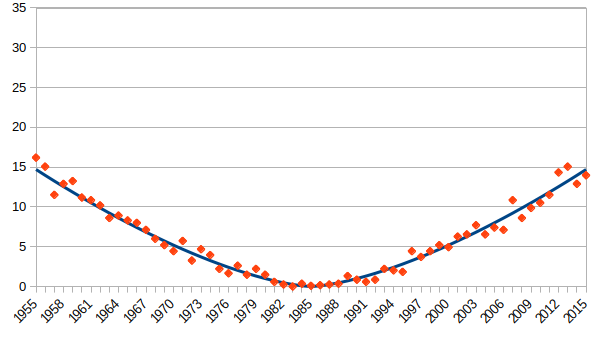In this case, the arithmetic mean of the errors in module is the divergence. For this function, we would obtain a deviation of 0,643979. Still insufficient for our criteria to change the model that explains the universe.

On the other hand, we can imagine that the time machine was invented and in this universe there were great variations resulting from the temporal movement. This consequently generated a divergence greater than 1, leading to the need to change the current model used to represent the universe.

In the next example, I took the function f (x) = |x|3/2 to simulate the current universe and a random y between -0,5 and 0,5. The divergent points are then represented in red and described by f(x) = |x+y|. Consequently they are better suited to a universe g (x) = |x| (pink curve) than the universe f(x) = |x|3/2 (blue curve). This in the context of Steins; Gate would lead to a change in the model adopted for the universe and a restructuring in the course of its planned events. The new red dots generate a module error in relation to the blue curve of 3,022655. However, an error in module in relation to the pink curve of 0,259016.To complete the analysis of how this concept works in the theory of time travel, know that it is sufficient to explain other fictional scenarios, such as the trilogy “Back to the future”. In a simple way I will tell you about these films based only on time travel, in the case of universes that occur in the same year more than once, we will refer to them with the addition of a capital letter. The trilogy begins in 1985A when Doctor Emmett Brown invents a time machine and sends his dog (called Einstein) to 1 minute from the future (1985A).

2. Marty McFly travels to 1955;

3. Marty McFly travels to 1985B;

4. Dr. Brown travels to 2015A;

5. Dr. Brown travels to 1985B;

6. Dr. Brown, Marty McFly and Jennifer Parker travel to 2015B;

7. Biff Tannen travels to 1955;

8. Biff Tannen travels to 2015B;

9. Dr. Brown, Marty McFly and Jennifer Parker travel to 1985C;

10. Dr. Brown travels to 1855;

11. Marty McFly travels to 1855;

12. Marty McFly travels to 1985D and meets Jennifer Parker from 1985C;

13. Dr. Brown and company travel to an unknown future;

14. Dr. Brown and company travel to 1985D;

15. Dr. Brown and company travel to an unknown past.In the figure above I present a flow chart of how time travel occurs in this trilogy. If you found it confusing to analyze this plot from a temporal point of view, don’t worry, this is reason for long discussions even in the series The Big Bang Theory, when Sheldon and his friends seek to explain some temporal differences in this trilogy (episode 5 of season 8 , “The Attenuation of the Focus”).

In this flowchart, the pink line from 1955 to 2015B represents Biff Tannen’s time travel. Here he returns to 2015B, which is still the universe adjusted to the 1985B events. Only when Dr. Brown, Marty McFly and Jennifer Parker travel to 1985, do they enter the universe adjusted to the changes made on the 8th trip, taking them to 1985C.

Thus, there are many questions about the influence of Biff Tannen in 1955, who spawned 1985C, should also spawn an immediate 2015C for when he returns. But with the divergence meter concept we have:

1. Dr. Brown built his time machine in 1985, as early as 2015B he would also be able to accomplish this feat;

2. Regardless of the destination that the original time machine came to, it would not prevent Dr. Brown from traveling in time;

3. The function of the universe when Biff Tannen traveled was based on results between 1955 and 2015B (60 years old);

4. When Dr. Brown returned, the function of the universe was recalculated over an interval of 30 years (1955-1985), and in this interval, the influence of Biff Tannen was enough for a change in the model of the universe, leading them to 1985C.

In the figure below I present the atypical event generated by the influence of Biff Tannen in 1955 for two different time intervals (60 and 30 years) and their respective measures of divergence.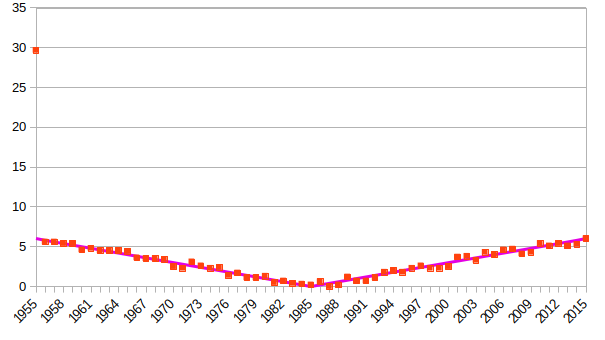Biff Tannen’s influence appears in 1955, but the average module errors in this case is 0,676686.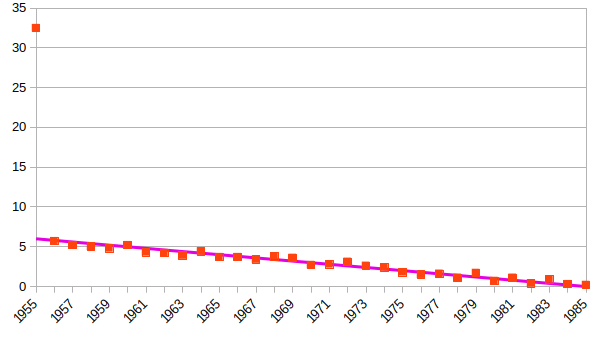For half the range, the divergence is now 1,131543.

Another interesting case that can be explained by the concept of divergence within this same trilogy would be the fact that Jennifer Parker stayed in 1985C and after the events of 1855 that led to a 1985D, the same Jennifer Parker from 1985C was there. In this case, this character existed in 1985C and without a device like a time machine, it was transported to 1985D during the change of the functions that shape the universe (events that generated divergences greater than 1). One explanation would be for her to be a time traveler, and for this reason a fixed result in the model of the universe, demanding that the model accept that value without changing.

It would be like an accurate, correct, but atypical measurement in an experiment that works very well within a model in which this result does not fit. We know that the model explains practically the whole phenomenon with the exception of this result, which would be explained by another function. However, it is not interesting to modify the model to fit this result, and to misfit all others. The solution in this case is to accept the atypical result and continue with the function.

The red dots are the temporal divergences in this universe, and Jennifer Parker is a divergence from an unknown universe (due to the time factor). Although a result is atypical, the model describes all other events well, justifying its use even if there is a divergence clearly distant from the curve.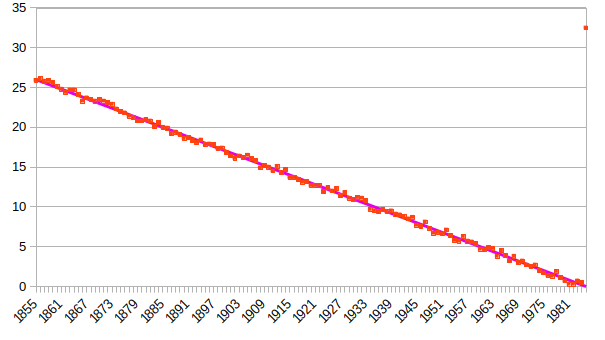The existence of Jennifer Parker appears as an atypical result in 1985, but the average of the errors in module in this case is 0,505174.